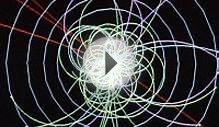# Higgs field and gravity

May 2, 2023One of the questions I get most often from my readers is this:

• Since gravity pulls on things proportional to their mass, and since the Higgs field is responsible for giving everything its mass, there obviously must be a deep connection between the Higgs and gravity… right?

It’s a very reasonable guess, but — it turns out to be completely wrong. The problem is that this statement combines a 17th century notion of gravity, long ago revised, with an overly simplified version of a late-20th century notion of where masses of various particles comes from. I’ve finally produced the Higgs FAQ version 2.0, intended for non-experts with little background in the subject, and as part of that, I’ve answered this question. But since the question is so common, I thought I’d also put the answer in a post of its own.

As preface, let me bring out my professorial training and correct the question above with a red pen:

• Since gravity pulls on things proportional to their mass to a combination of their energy and momentum, and since the Higgs field is responsible of giving everything not everything, just the known elementary particles excepting the Higgs particle itself its mass, there obviously must be a deep connection between the Higgs and gravity… right? wrong.

Now let me explain these corrections one by one.

When you first learn about gravity in school, you learn Newton’s law: that the force of gravity between two objects, one of mass M1 and one of mass M2, has a strength proportional to the product M1 M2.

But that was true before Einstein. It turns out that Newton’s law needs to be revised: the Einsteinian statement of the law is (roughly) that for two objects that are slow-moving (i.e. their speed relative to one another is much less than c, the speed of light) and have energy E1 and E2, the gravitational force between them has a strength proportional to the product E1 E2.

How are these two statements, the Newtonian and the Einsteinian, consistent? They are consistent because Einstein and his followers established that for any ordinary object, the relation between its energy E, momentum p and mass M [sometimes called “rest mass”, but just called `mass’ by particle physicists] is

For a slow-moving object, p ≈ Mv (where v is the object’s velocity) and pc ≈ Mvc is much smaller than Mc2. And therefore